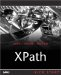Creating Sequence Expressions

As we've seen in Chapter 7, you can create sequence expressions with the comma (,) operator. In XPath 2.0, the comma operator evaluates each of its operands and joins the results into a sequence.

You can use empty parentheses to indicate an empty sequence. Also note that sequences can never be nestedfor example, combining the values 4, (5, 6), into a single sequence results in the sequence (4, 5, 6). Sequences can contain nodes or atomic values, or a mix.

Sequences may contain duplicate values or nodes. When you create a new sequence by concatenating two or more input sequences, the new sequence contains all the items in the original sequences.

We'll start our work on sequence expressions by seeing how to create sequences with them.

Creating Sequences

Here are a few examples; this sequence expression creates a sequence of six integers:

(9, 10, 11, 12, 13, 14)

In this case, we're creating a new sequence from the sequences 1, (2, 3), () that is, an empty sequenceand (4, 5, 6):

(1, (2, 3), (), 4, 5, 6))

This expression gives you the following sequence:

1, 2, 3, 4, 5, 6

Here's how you could create a sequence made up of all the < name > children of the context node, followed by all the <mass> children:

(name, mass)

You can also use variables or other expressions when creating sequences, like this:

(\$temperature, 3 * 6)

If \$temperature holds 68, this example gives you this sequence:

(68, 18)

You can also use the range operator, as mentioned in Chapter 7, to create a sequence of integers. Here's an example:

(1 to 5)

This sequence expression creates the following sequence:

(1, 2, 3, 4, 5)

You can also use the range operator inside a sequence expression, like this:

(1, 2 to 5)

Here's the result:

1, 2, 3, 4, 5

What if you were to make the start and end of the range the same value by mistake? For example, how is XPath 2.0 supposed to handle this?:

9999 to 9999

In this case, the result is a singleton sequence with just one item, 9999.

Combining Sequences

XPath 2.0 also gives you a set of operators to work with sequences, as we saw in the overview in Chapter 7. We'll take a look at these operators union , intersect , and except which create sequence expressions, next .

EXCLUDING DUPLICATES

The union , intersect , and except operators return their results as sequences in document order, without any duplicate items in those sequences.

The union Operator

The union operator takes two node sequences as operands and returns a sequence containing all the nodes that occur in either of the original sequences. This operator works something like the logical or operator in other languages. The union operator is identical to the operator.

Here are a few examples, where a , b , c , d , and e are nodes:

a, b, union c, d, e

This expression gives you

a, b, c, d, e

On the other hand, duplicate items do not appear in the resulting sequence. This expression

a, b, c union c, d, e

gives you

a, b, c, d, e
The intersect Operator

The intersect operator takes two node sequences as operands and returns a sequence containing all the nodes that occur in both operands. This operator works like the logical and operator in other languages.

Here are a few examples; in this expression, both the nodes b and c are common to both sequence operands:

a, b, c intersect b, c, d

And here's the result:

b, c

This sequence combination expression

d, e, f intersect b, c, d

gives you just a singleton sequence:

d

If you try to find the intersection of two sequences that have no items in common, like this:

a, b intersect c, d

the result will be an empty sequence, () .

The except Operator

The except operator takes two node sequences as operands and returns a sequence containing all the nodes that occur in the first operand but not in the second operand.

Here's an example:

a, b, c except c

gives you

a, b

Here's another example:

a, b, c, d except c, d, e

gives you

a, b

This expression

a, b, c except d, e, f

gives you

a, b, c

FUNCTIONS THAT SUPPORT INDEXED ACCESS

Besides the sequence operators we're discussing here that are evaluated as XPath expressions, there's also a set of functions that support indexed access to items or subsequences of a sequence, indexed insertion or removal of items in a sequence, and removal of duplicate values or nodes from a sequence. They're coming up in Chapter 12, "XPath 2.0 Node and Sequence Functions."

And this expression

a, b, c except a, b, c, d, e

gives you an empty sequence, () .XPath Kick Start: Navigating XML with XPath 1.0 and 2.0
ISBN: 0672324113
EAN: 2147483647
Year: 2002
Pages: 131

Similar book on Amazon